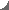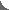Contact arthur.gretton@gmail.com
Gatsby Computational Neuroscience Unit
Sainsbury Wellcome Centre
25 Howland Street
London W1T 4JG UKMMD GAN training and evaluation

New gradient penalty method (June 2018) with state-of-the-art performance. Code
Earlier ICLR 2018 Code

• MMD for criticising generative models
Used to evaluate the output and to train generative adversarial networks, from ICLR 2017
Code

• Linear-time Stein test for goodness-of-fit
An adaptive linear time test for goodness-of-fit of models, from NIPS 2017. Code
Earlier quadratic-time kernel Stein discrepancy (ICML 2016) Code

• Fast Independence Testing with Analytic Representations of Probability Measures
Adaptive independence test with a cost linear in the sample size, from ICML 2017.
Code

• Fast Two-Sample Testing with Analytic Representations of Probability Measures
A class of powerful nonparametric two-sample tests with a cost linear in the sample size.
Earlier NIPS 2015 code (random features)

Infinite dimensional exponential families and adaptive HMC

• Efficient density estimator, infinite dimensional exponential family
Nystrom approximation to infinite dimensional exponential family with convergence guarantees. AISTATS 2018. Code

• Conditional infinite exponential family
Conditional infinite exponential family, learns conditional density models which can be sampled by HMC. AISTATS 2018. Code

• Gradient-free Hamiltonian Monte Carlo with Efficient Kernel Exponential Families
A gradient-free adaptive MCMC algorithm based on Hamiltonian Monte Carlo (HMC). On target densities where classical HMC is not an option due to intractable gradients, KMC adaptively learns the target's gradient structure from the sample path, by fitting an exponential family model in a Reproducing Kernel Hilbert Space. NIPS 2015.
Code

A kernel adaptive Metropolis-Hastings algorithm for sampling from a target distribution with strongly nonlinear support. The algorithm embeds the trajectory of the Markov chain into a reproducing kernel Hilbert space (RKHS), such that the feature space covariance of the samples informs the choice of proposal. In this way, the proposal distribution in the original space adapts to the local covariance structure of the target. ICML 2014.
Code

Hypothesis testing for time series

• Wild bootstrap tests for time series
Statistical tests for random processes, providing two-sample tests based on MMD (for the marginal distributions of the random processes) and independence tests based on HSIC. The procedure uses a wild bootstrap approach (see NIPS 2014 paper for details).
Code

• Independence test for time series
A test of independence for two random processes, based on the Hilbert Schmidt Independence Criterion (HSIC). The procedure uses a bootstrap approach designed to work in the case of random processes (the bootstrap for the i.i.d. case would return too many false positives).From ICML 2014.
Code

Distribution regression and message passing

• Distribution to real regression
Regression from distributions to reals, by embedding the distributions to a reproducing kernel Hilbert space, and learning a ridge regressor from the embeddings to the outputs. The method gives state-of-the-art results on (i) supervised entropy learning and (ii) the prediction problem of aerosol optical depth based on satellite images.
Code

• Kernel-Based Just-In-Time Learning for Passing Expectation Propagation Messages
A fast, online algorithm for nonparametric learning of EP message updates.
Code

• Kernel Belief Propagation
A nonparametric approach to learning and inference on trees and graphs with loops, using a kernelized message passing algorithm.
Code

Hypothesis testing using MMD, HSIC, and more

• Kernel goodness-of-fit test
A quadratic-time goodness-of-fit test using the kernel Stein discrepancy (ICML 2016)
Code

• Non-parametric, low variance kernel two-sample tests
A family of maximum mean discrepancy (MMD) kernel two-sample tests. A hyperparameter controls the tradeoff between sample complexity and computational time, avoiding the quadratic number of kernel evaluations and the complex null-hypothesis approximation required by tests relying on U-statistics.
Code

• Three variable interaction tests
Kernel nonparametric tests for Lancaster three-variable interaction and for total independence. The resulting test statistics are straightforward to compute, and the tests are consistent against all alternatives for a large family of reproducing kernels. The Lancaster test is especially useful where two independent causes individually have weak inﬂuence on a third dependent variable, but their combined effect has a strong inﬂuence (e.g in ﬁnding structure in directed graphical models).
Code

• Optimal kernel choice for large-scale two-sample tests
An optimal kernel selection procedure for the kernel two-sample test. For a given test level (an upper bound on the probability of making a Type I error), the kernel is chosen so as to maximize the test power, and minimize the probability of making a Type II error. The test procedure has cost linear in the sample size, making it suited to data streams.
Code

• Kernel Two-Sample Test (updated March 2012)
A kernel method to perform a statistical test of whether two samples are from different distributions. This test can be applied to high dimensional data, as well as to non-vectorial data such as graphs (i.e., wherever kernels provide a similarity measure).
Code

• Statistical Independence Tests
Three different statistical tests of whether two random variables are independent. The test statistics are: a kernel statistic (the Hilbert-Schmidt Independence Criterion), an L1 statistic, and a log-likelihood statistic (the mutual information).
Code

Covariate shift correction, taxonomic structure learning, independent component analysis

• Taxonomic Prediction with Tree-Structured Covariances
Data-derived taxonomies are used in a structured prediction framework. Structured prediction in this case is multi-class categorization with the assumption that categories are taxonomically related. The taxonomies are learned from data using the Hilbert-Schmidt Independence Criterion (HSIC).
Code

• Fast Kernel ICA
Kernel ICA uses kernel measures of statistical independence to separate linearly mixed sources. We have made this process much faster by using an approximate Newton-like method on the special orthogonal group to perform the optimisation.
Code

• Nonlinear directed acyclic structure learning
This algorithm learns the structure of a directed graphical model from data, combining a PC style search using nonparametric (kernel) measures of conditional dependence with local searches for additive noise models.
Code

• Covariate Shift Correction
Given sets of observations of training and test data, we reweight the training data such that its distribution more closely matches that of the test data. We achieve this goal by matching covariate distributions between training and test sets in a reproducing kernel Hilbert space.
Code